# Graph

5 de Jan de 2017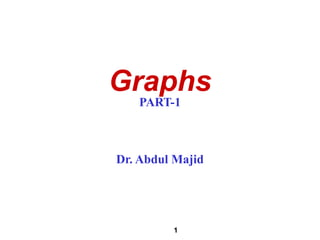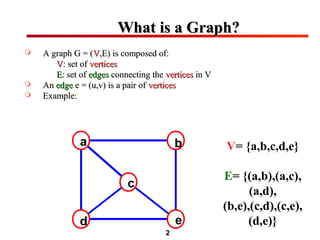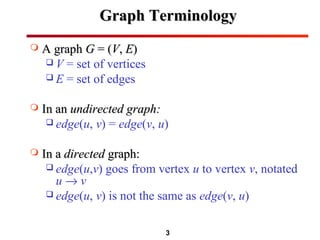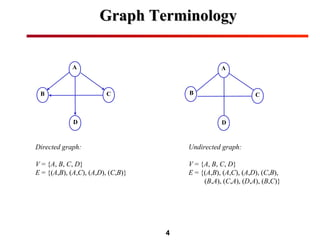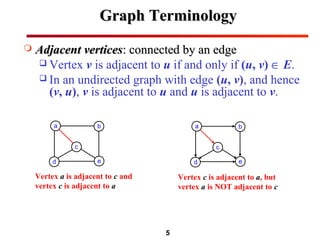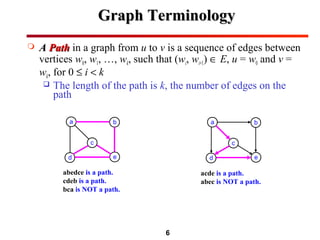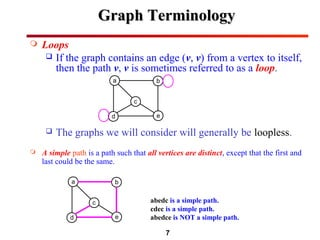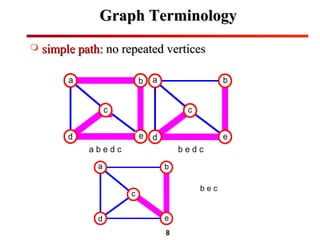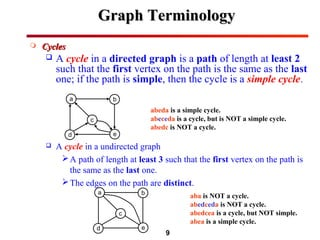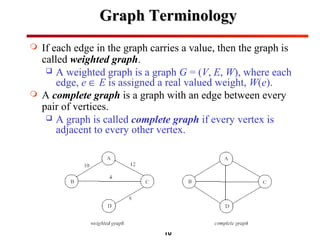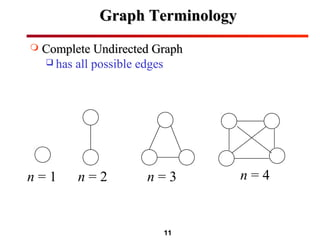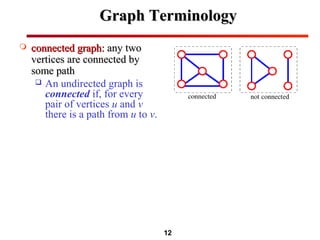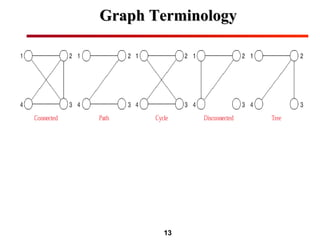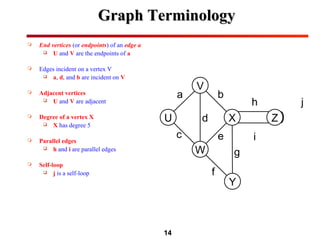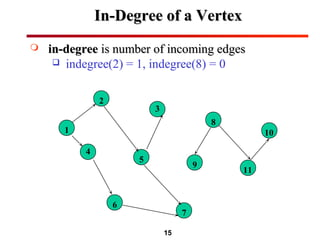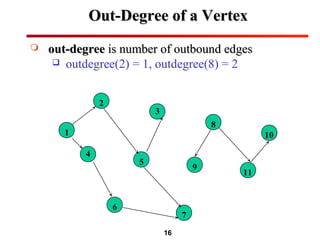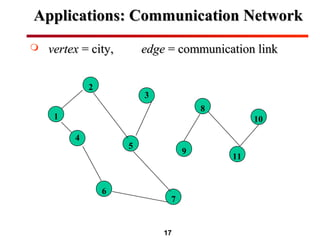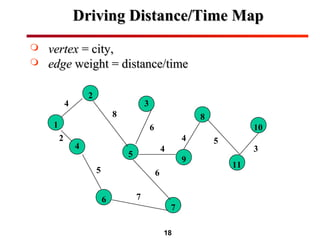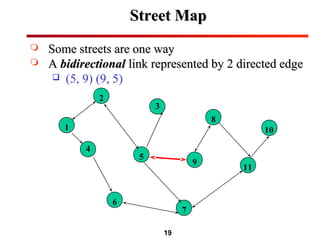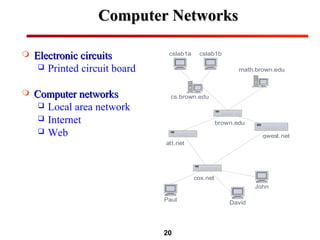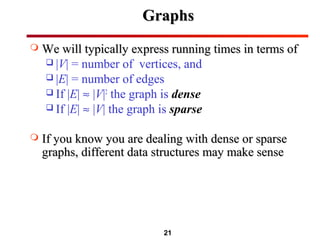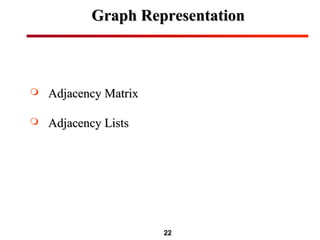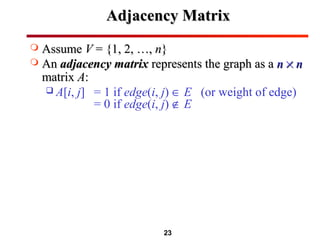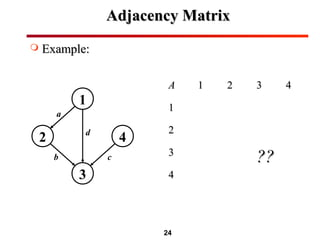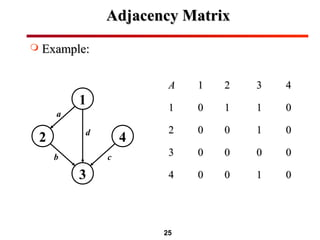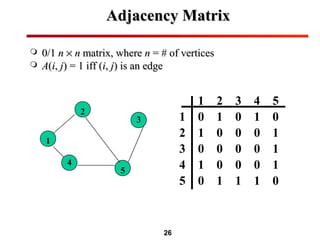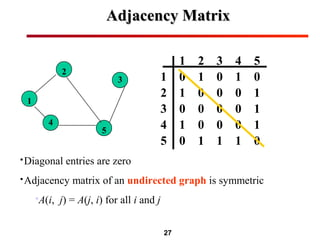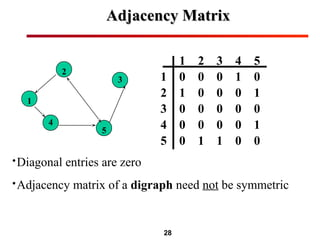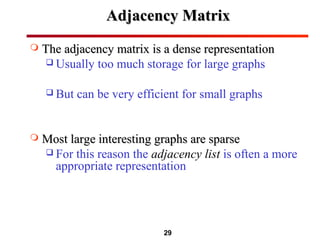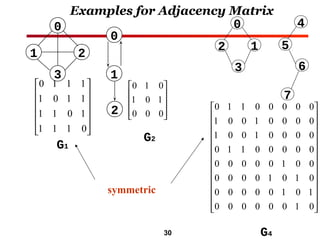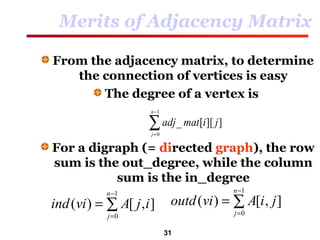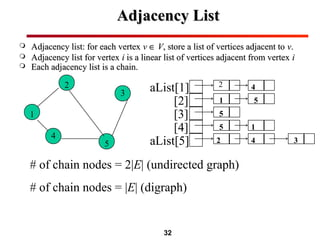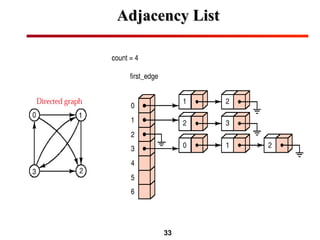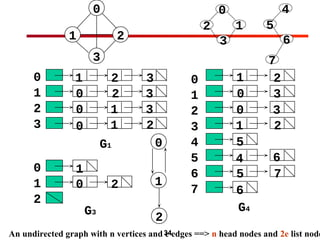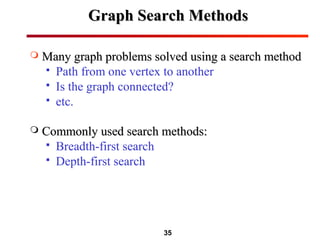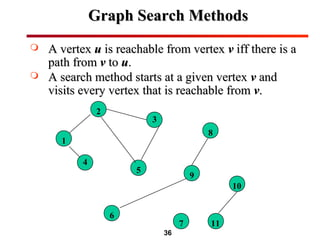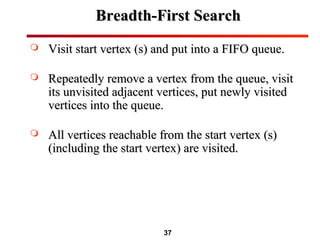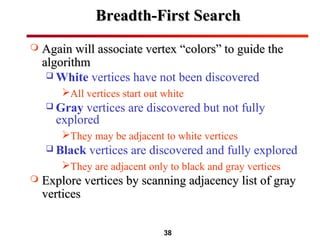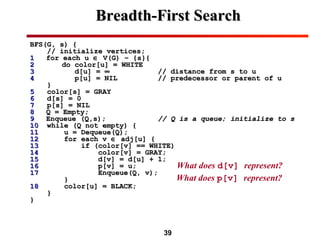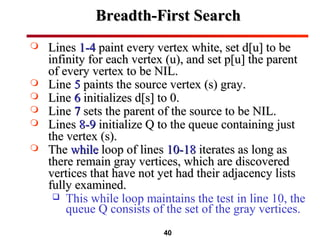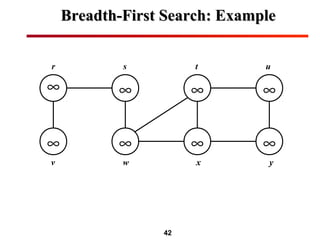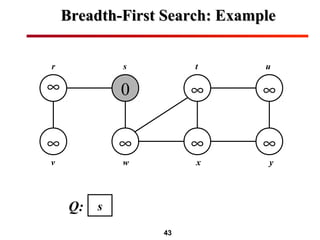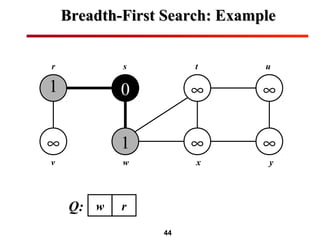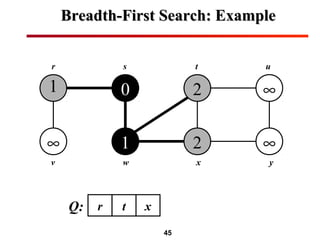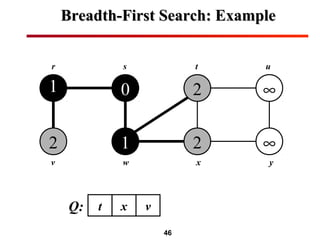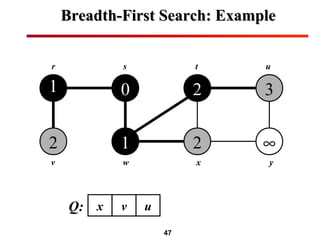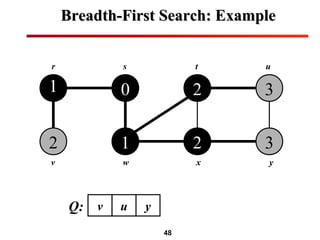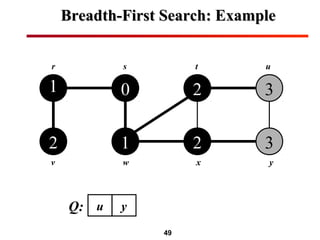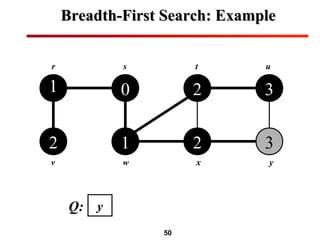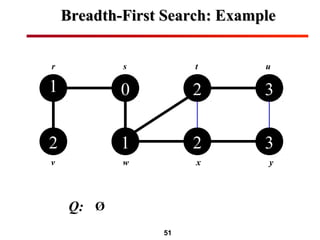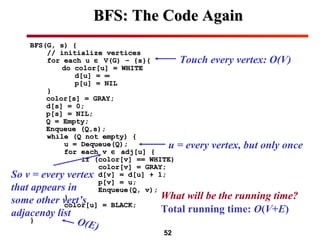1 de 52

### Graph

• 1. 1 Graphs PART-1 Dr. Abdul Majid
• 2. 2 What is a Graph?What is a Graph?  A graph G = (A graph G = (VV,E) is composed of:,E) is composed of: VV: set of: set of verticesvertices EE: set of: set of edgesedges connecting theconnecting the verticesvertices in Vin V  AnAn edgeedge e = (u,v) is a pair ofe = (u,v) is a pair of verticesvertices  Example:Example: a b c d e V= {a,b,c,d,e} E= {(a,b),(a,c), (a,d), (b,e),(c,d),(c,e), (d,e)}
• 3. 3 Graph TerminologyGraph Terminology  A graphA graph GG = (= (VV,, EE))  V = set of vertices  E = set of edges  In anIn an undirected graph:undirected graph:  edge(u, v) = edge(v, u)  In aIn a directeddirected graph:graph:  edge(u,v) goes from vertex u to vertex v, notated u → v  edge(u, v) is not the same as edge(v, u)
• 4. 4 Graph TerminologyGraph Terminology Directed graph: V = {A, B, C, D} E = {(A,B), (A,C), (A,D), (C,B)} A C D B Undirected graph: V = {A, B, C, D} E = {(A,B), (A,C), (A,D), (C,B), (B,A), (C,A), (D,A), (B,C)} A C D B
• 5. 5 Graph TerminologyGraph Terminology  Adjacent verticesAdjacent vertices: connected by an edge: connected by an edge  Vertex v is adjacent to u if and only if (u, v) ∈ E.  In an undirected graph with edge (u, v), and hence (v, u), v is adjacent to u and u is adjacent to v. a b d e c a b d e c Vertex a is adjacent to c and vertex c is adjacent to a Vertex c is adjacent to a, but vertex a is NOT adjacent to c
• 6. 6  AA PathPath in a graph from u to v is a sequence of edges between vertices w0, w1, …, wk, such that (wi, wi+1) ∈ E, u = w0 and v = wk, for 0 ≤ i < k  The length of the path is k, the number of edges on the path a b d e c a b d e c abedce is a path. cdeb is a path. bca is NOT a path. acde is a path. abec is NOT a path. Graph TerminologyGraph Terminology
• 7. 7  Loops  If the graph contains an edge (v, v) from a vertex to itself, then the path v, v is sometimes referred to as a loop.  The graphs we will consider will generally be loopless.  A simple path is a path such that all vertices are distinct, except that the first and last could be the same. a b d e c abedc is a simple path. cdec is a simple path. abedce is NOT a simple path. a b d e c Graph TerminologyGraph Terminology
• 8. 8 Graph TerminologyGraph Terminology  simple path:simple path: no repeated verticesno repeated vertices
• 9. 9  CyclesCycles  A cycle in a directed graph is a path of length at least 2 such that the first vertex on the path is the same as the last one; if the path is simple, then the cycle is a simple cycle.  A cycle in a undirected graph A path of length at least 3 such that the first vertex on the path is the same as the last one. The edges on the path are distinct. abeda is a simple cycle. abeceda is a cycle, but is NOT a simple cycle. abedc is NOT a cycle. a b d e c a b d e c aba is NOT a cycle. abedceda is NOT a cycle. abedcea is a cycle, but NOT simple. abea is a simple cycle. Graph TerminologyGraph Terminology
• 10. 10 Graph TerminologyGraph Terminology  If each edge in the graph carries a value, then the graph is called weighted graph.  A weighted graph is a graph G = (V, E, W), where each edge, e ∈ E is assigned a real valued weight, W(e).  A complete graph is a graph with an edge between every pair of vertices.  A graph is called complete graph if every vertex is adjacent to every other vertex.
• 11. 11 Graph TerminologyGraph Terminology  Complete Undirected GraphComplete Undirected Graph  has all possible edges n = 1 n = 2 n = 3 n = 4
• 12. 12 Graph TerminologyGraph Terminology  connected graph:connected graph: any twoany two vertices are connected byvertices are connected by some pathsome path  An undirected graph is connected if, for every pair of vertices u and v there is a path from u to v.
• 13. 13 Graph TerminologyGraph Terminology
• 14. 14  End vertices (or endpoints) of an edge a  U and V are the endpoints of a  Edges incident on a vertex V  a, d, and b are incident on V  Adjacent vertices  U and V are adjacent  Degree of a vertex X  X has degree 5  Parallel edges  h and i are parallel edges  Self-loop  j is a self-loop XU V W Z Y a c b e d f g h i j Graph TerminologyGraph Terminology
• 15. 15 In-Degree of a VertexIn-Degree of a Vertex  in-degreein-degree is number of incoming edgesis number of incoming edges  indegree(2) = 1, indegree(8) = 0 2 3 8 101 4 5 9 11 6 7
• 16. 16 Out-Degree of a VertexOut-Degree of a Vertex  out-degreeout-degree is number of outbound edgesis number of outbound edges  outdegree(2) = 1, outdegree(8) = 2 2 3 8 101 4 5 9 11 6 7
• 17. 17 Applications: Communication NetworkApplications: Communication Network  vertexvertex = city,= city, edgeedge = communication link= communication link 2 3 8 101 4 5 9 11 6 7
• 18. 18 Driving Distance/Time MapDriving Distance/Time Map  vertexvertex = city,= city,  edgeedge weight = distance/timeweight = distance/time 2 3 8 101 4 5 9 11 6 7 4 8 6 6 7 5 2 4 4 5 3
• 19. 19 Street MapStreet Map  Some streets are one waySome streets are one way  AA bidirectionalbidirectional link represented by 2 directed edgelink represented by 2 directed edge  (5, 9) (9, 5) 2 3 8 101 4 5 9 11 6 7
• 20. 20 John David Paul brown.edu cox.net cs.brown.edu att.net qwest.net math.brown.edu cslab1bcslab1a Electronic circuitsElectronic circuits  Printed circuit board  Computer networksComputer networks  Local area network  Internet  Web Computer NetworksComputer Networks
• 21. 21 GraphsGraphs  We will typically express running times in terms ofWe will typically express running times in terms of  |V| = number of vertices, and  |E| = number of edges  If |E| ≈ |V|2 the graph is dense  If |E| ≈ |V| the graph is sparse  If you know you are dealing with dense or sparseIf you know you are dealing with dense or sparse graphs, different data structures may make sensegraphs, different data structures may make sense
• 23. 23 Adjacency MatrixAdjacency Matrix  AssumeAssume VV = {1, 2, …,= {1, 2, …, nn}}  AnAn adjacency matrixadjacency matrix represents the graph as arepresents the graph as a nn ×× nn matrixmatrix AA::  A[i, j] = 1 if edge(i, j) ∈ E (or weight of edge) = 0 if edge(i, j) ∉ E
• 24. 24 Adjacency MatrixAdjacency Matrix  Example:Example: 1 2 4 3 a d b c AA 11 22 33 44 11 22 33 ???? 44
• 25. 25 Adjacency MatrixAdjacency Matrix  Example:Example: 1 2 4 3 a d b c AA 11 22 33 44 11 00 11 11 00 22 00 00 11 00 33 00 00 00 00 44 00 00 11 00
• 26. 26 Adjacency MatrixAdjacency Matrix  0/10/1 nn ×× nn matrix, wherematrix, where nn = # of vertices= # of vertices  AA((ii,, jj) = 1 iff () = 1 iff (ii,, jj) is an edge) is an edge 2 3 1 4 5 1 2 3 4 5 1 2 3 4 5 0 1 0 1 0 1 0 0 0 1 0 0 0 0 1 1 0 0 0 1 0 1 1 1 0
• 27. 27 Adjacency MatrixAdjacency Matrix 2 3 1 4 5 1 2 3 4 5 1 2 3 4 5 0 1 0 1 0 1 0 0 0 1 0 0 0 0 1 1 0 0 0 1 0 1 1 1 0 •Diagonal entries are zero •Adjacency matrix of an undirected graph is symmetric A(i, j) = A(j, i) for all i and j
• 28. 28 Adjacency MatrixAdjacency Matrix 2 3 1 4 5 1 2 3 4 5 1 2 3 4 5 0 0 0 1 0 1 0 0 0 1 0 0 0 0 0 0 0 0 0 1 0 1 1 0 0 •Diagonal entries are zero •Adjacency matrix of a digraph need not be symmetric
• 29. 29 Adjacency MatrixAdjacency Matrix  The adjacency matrix is a dense representationThe adjacency matrix is a dense representation  Usually too much storage for large graphs  But can be very efficient for small graphs  Most large interesting graphs are sparseMost large interesting graphs are sparse  For this reason the adjacency list is often a more appropriate representation
• 30. 30 Examples for Adjacency Matrix 0 1 1 1 1 0 1 1 1 1 0 1 1 1 1 0             0 1 0 1 0 0 0 1 0           0 1 1 0 0 0 0 0 1 0 0 1 0 0 0 0 1 0 0 1 0 0 0 0 0 1 1 0 0 0 0 0 0 0 0 0 0 1 0 0 0 0 0 0 1 0 1 0 0 0 0 0 0 1 0 1 0 0 0 0 0 0 1 0                           G1 G2 G4 0 1 2 3 0 1 2 1 0 2 3 4 5 6 7 symmetric
• 31. 31 Merits of Adjacency Matrix From the adjacency matrix, to determine the connection of vertices is easy The degree of a vertex is For a digraph (= directed graph), the row sum is the out_degree, while the column sum is the in_degree adj mat i j j n _ [ ][ ] = − ∑0 1 ind vi A j i j n ( ) [ , ]= = − ∑ 0 1 outd vi A i j j n ( ) [ , ]= = − ∑ 0 1
• 34. 34 0 1 2 3 0 1 2 0 1 2 3 4 5 6 7 1 2 3 0 2 3 0 1 3 0 1 2 G1 1 0 2 G3 1 2 0 3 0 3 1 2 5 4 6 5 7 6 G4 0 1 2 3 0 1 2 1 0 2 3 4 5 6 7 An undirected graph with n vertices and e edges ==> n head nodes and 2e list node
• 35. 35 Graph Search MethodsGraph Search Methods  Many graph problems solved using a search methodMany graph problems solved using a search method  Path from one vertex to another  Is the graph connected?  etc.  Commonly used search methods:Commonly used search methods:  Breadth-first search  Depth-first search
• 36. 36 Graph Search MethodsGraph Search Methods  A vertexA vertex uu is reachable from vertexis reachable from vertex vv iff there is aiff there is a path frompath from vv toto uu..  A search method starts at a given vertexA search method starts at a given vertex vv andand visits every vertex that is reachable fromvisits every vertex that is reachable from vv.. 2 3 8 10 1 4 5 9 11 6 7
• 37. 37 Breadth-First SearchBreadth-First Search  Visit start vertex (s) and put into a FIFO queue.Visit start vertex (s) and put into a FIFO queue.  Repeatedly remove a vertex from the queue, visitRepeatedly remove a vertex from the queue, visit its unvisited adjacent vertices, put newly visitedits unvisited adjacent vertices, put newly visited vertices into the queue.vertices into the queue.  All vertices reachable from the start vertex (s)All vertices reachable from the start vertex (s) (including the start vertex) are visited.(including the start vertex) are visited.
• 38. 38 Breadth-First SearchBreadth-First Search  Again will associate vertex “colors” to guide theAgain will associate vertex “colors” to guide the algorithmalgorithm  White vertices have not been discovered All vertices start out white  Gray vertices are discovered but not fully explored They may be adjacent to white vertices  Black vertices are discovered and fully explored They are adjacent only to black and gray vertices  Explore vertices by scanning adjacency list of grayExplore vertices by scanning adjacency list of gray verticesvertices
• 39. 39 Breadth-First SearchBreadth-First Search BFS(G, s) {BFS(G, s) { // initialize vertices;// initialize vertices; 11 for each ufor each u ∈∈ V(G) – {s}{V(G) – {s}{ 22 do color[u] = WHITEdo color[u] = WHITE 33 d[u] =d[u] = ∞∞ // distance from s to u// distance from s to u 44 p[u] = NILp[u] = NIL // predecessor or parent of u// predecessor or parent of u }} 55 color[s] = GRAYcolor[s] = GRAY 66 d[s] = 0d[s] = 0 77 p[s] = NILp[s] = NIL 88 Q = Empty;Q = Empty; 99 Enqueue (Q,s);Enqueue (Q,s); // Q is a queue; initialize to s// Q is a queue; initialize to s 1010 while (Q not empty) {while (Q not empty) { 1111 u = Dequeue(Q);u = Dequeue(Q); 1212 for each vfor each v ∈∈ adj[u] {adj[u] { 1313 if (color[v] == WHITE)if (color[v] == WHITE) 1414 color[v] = GRAY;color[v] = GRAY; 1515 d[v] = d[u] + 1;d[v] = d[u] + 1; 1616 p[v] = u;p[v] = u; 1717 Enqueue(Q, v);Enqueue(Q, v); }} 1818 color[u] = BLACK;color[u] = BLACK; }} }} What does p[v] represent? What does d[v] represent?
• 40. 40 Breadth-First SearchBreadth-First Search  LinesLines 1-41-4 paint every vertex white, set d[u] to bepaint every vertex white, set d[u] to be infinity for each vertex (u), and set p[u] the parentinfinity for each vertex (u), and set p[u] the parent of every vertex to be NIL.of every vertex to be NIL.  LineLine 55 paints the source vertex (s) gray.paints the source vertex (s) gray.  LineLine 66 initializes d[s] to 0.initializes d[s] to 0.  LineLine 77 sets the parent of the source to be NIL.sets the parent of the source to be NIL.  LinesLines 8-98-9 initialize Q to the queue containing justinitialize Q to the queue containing just the vertex (s).the vertex (s).  TheThe whilewhile loop of linesloop of lines 10-1810-18 iterates as long asiterates as long as there remain gray vertices, which are discoveredthere remain gray vertices, which are discovered vertices that have not yet had their adjacency listsvertices that have not yet had their adjacency lists fully examined.fully examined.  This while loop maintains the test in line 10, the queue Q consists of the set of the gray vertices.
• 41. 41 Breadth-First SearchBreadth-First Search  Prior to the first iteration in linePrior to the first iteration in line 1010, the only gray vertex, and, the only gray vertex, and the only vertex in Q, is the source vertex (s).the only vertex in Q, is the source vertex (s).  LineLine 1111 determines the gray vertex (u) at the head of the queuedetermines the gray vertex (u) at the head of the queue Q and removes it from Q.Q and removes it from Q.  TheThe forfor loop of linesloop of lines 12-1712-17 considers each vertex (v) in theconsiders each vertex (v) in the adjacency list of (u).adjacency list of (u).  If (v) is white, then it has not yet been discovered, and theIf (v) is white, then it has not yet been discovered, and the algorithm discovers it by executing linesalgorithm discovers it by executing lines 14-1714-17..  It is first grayed, and its distance d[v] is set to d[u]+1.  Then, u is recorded as its parent.  Finally, it is placed at the tail of the queue Q.  When all the vertices on (u’s) adjacency list have beenWhen all the vertices on (u’s) adjacency list have been examined, u is blackened in lineexamined, u is blackened in line 1818..
• 42. 42 Breadth-First Search: ExampleBreadth-First Search: Example ∞ ∞ ∞ ∞ ∞ ∞ ∞ ∞ r s t u v w x y
• 43. 43 Breadth-First Search: ExampleBreadth-First Search: Example ∞ ∞ 0 ∞ ∞ ∞ ∞ ∞ r s t u v w x y sQ:
• 44. 44 Breadth-First Search: ExampleBreadth-First Search: Example 1 ∞ 0 1 ∞ ∞ ∞ ∞ r s t u v w x y wQ: r
• 45. 45 Breadth-First Search: ExampleBreadth-First Search: Example 1 ∞ 0 1 2 2 ∞ ∞ r s t u v w x y rQ: t x
• 46. 46 Breadth-First Search: ExampleBreadth-First Search: Example 1 2 0 1 2 2 ∞ ∞ r s t u v w x y Q: t x v
• 47. 47 Breadth-First Search: ExampleBreadth-First Search: Example 1 2 0 1 2 2 3 ∞ r s t u v w x y Q: x v u
• 48. 48 Breadth-First Search: ExampleBreadth-First Search: Example 1 2 0 1 2 2 3 3 r s t u v w x y Q: v u y
• 49. 49 Breadth-First Search: ExampleBreadth-First Search: Example 1 2 0 1 2 2 3 3 r s t u v w x y Q: u y
• 50. 50 Breadth-First Search: ExampleBreadth-First Search: Example 1 2 0 1 2 2 3 3 r s t u v w x y Q: y
• 51. 51 Breadth-First Search: ExampleBreadth-First Search: Example 1 2 0 1 2 2 3 3 r s t u v w x y Q: Ø
• 52. 52 BFS: The Code AgainBFS: The Code Again BFS(G, s) {BFS(G, s) { // initialize vertices// initialize vertices for each ufor each u ∈∈ V(G) – {s}{V(G) – {s}{ do color[u] = WHITEdo color[u] = WHITE d[u] =d[u] = ∞∞ p[u] = NILp[u] = NIL }} color[s] = GRAY;color[s] = GRAY; d[s] = 0;d[s] = 0; p[s] = NIL;p[s] = NIL; Q = Empty;Q = Empty; Enqueue (Q,s);Enqueue (Q,s); while (Q not empty) {while (Q not empty) { u = Dequeue(Q);u = Dequeue(Q); for each vfor each v ∈∈ adj[u] {adj[u] { if (color[v] == WHITE)if (color[v] == WHITE) color[v] = GRAY;color[v] = GRAY; d[v] = d[u] + 1;d[v] = d[u] + 1; p[v] = u;p[v] = u; Enqueue(Q, v);Enqueue(Q, v); }} color[u] = BLACK;color[u] = BLACK; }} }} What will be the running time? Touch every vertex: O(V) u = every vertex, but only once So v = every vertex that appears in some other vert’s adjacency list Total running time: O(V+E) O(E)

### Notas del editor

1. Internet connection. Vertices are computers. Send email from 1 to 7.
2. Array length n simply means we need an array with n spots. A direct implementation using a Java array would need n+1 spots, because spot 0 would not be utilized. However, by using spot 0 for vertex 1, spot 1 for vertex 2, and so on, we could get by with a Java array whose length is actually n.
3. Array length n simply means we need an array with n spots. A direct implementation using a Java array would need n+1 spots, because spot 0 would not be utilized. However, by using spot 0 for vertex 1, spot 1 for vertex 2, and so on, we could get by with a Java array whose length is actually n.